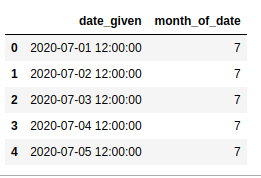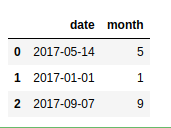# Get Month from date in Pandas – Python

Pandas has many inbuilt methods that can be used to extract the month from a given date that are being generated randomly using the random function or by using Timestamp function or that are transformed to date format using the to_datetimefunction. Let’s see few examples for better understanding.

Example 1

 `import` `pandas as pd ` ` `  ` `  `dti ``=` `pd.date_range(``'2020-07-01'``, periods ``=` `4``, freq ``=``'M'``) ` `print``(dti.month) `

Output:

```Int64Index([7, 8, 9, 10], dtype='int64')
```

Example 2

 `import` `pandas as pd ` `import` `numpy as np ` `import` `datetime ` ` `  `date1 ``=` `pd.Series(pd.date_range(``'2020-7-1 12:00:00'``, periods ``=` `5``)) ` `df ``=` `pd.DataFrame(``dict``(date_given ``=` `date1)) ` ` `  `df[``'month_of_date'``] ``=` `df[``'date_given'``].dt.month ` `df `

Output:Example 3

 `import` `pandas as pd ` `import` `datetime ` `import` `numpy as np ` ` `  ` `  `dates ``=``[``'14 / 05 / 2017'``, ``'2017'``, ``'07 / 09 / 2017'``] ` ` `  `frame ``=` `pd.to_datetime(dates, dayfirst ``=` `True``) ` `frame ``=` `pd.DataFrame([frame]).transpose() ` `frame[``'date'``]``=` `frame ` `frame[``'month'``]``=` `frame[``'date'``].dt.month ` `frame.drop(``0``, axis ``=` `1``, inplace ``=` `True``) ` ` `  `frame `

Output:Attention geek! Strengthen your foundations with the Python Programming Foundation Course and learn the basics.

To begin with, your interview preparations Enhance your Data Structures concepts with the Python DS Course.

My Personal Notes arrow_drop_upCheck out this Author's contributed articles.

If you like GeeksforGeeks and would like to contribute, you can also write an article using contribute.geeksforgeeks.org or mail your article to contribute@geeksforgeeks.org. See your article appearing on the GeeksforGeeks main page and help other Geeks.

Please Improve this article if you find anything incorrect by clicking on the "Improve Article" button below.

Article Tags :

Be the First to upvote.

Please write to us at contribute@geeksforgeeks.org to report any issue with the above content.